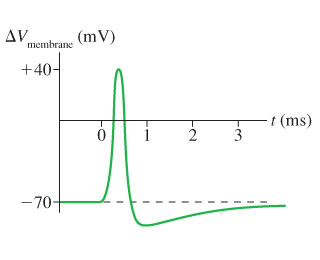# Problem: A 8.0 nm thick cell membrane undergoes an action potential that follows the curve in the figure.What is the strength of the electric field inside the membrane just before the action potential?

###### FREE Expert Solution

Electric field:

$\overline{){\mathbf{E}}{\mathbf{=}}{\mathbf{-}}\frac{\mathbf{∆}\mathbf{V}}{\mathbf{∆}\mathbf{x}}}$

Before action potential, ΔV = -70mV(10-3V/1mV) = -70 × 10-3 V###### Problem Details

A 8.0 nm thick cell membrane undergoes an action potential that follows the curve in the figure.What is the strength of the electric field inside the membrane just before the action potential?